# Java Tutorial - Java char

In Java, char stores characters. Java uses Unicode to represent characters. Unicode can represent all of the characters found in all human languages.

Java char is a 16-bit type.

The range of a char is `0` to `65,536`. There are no negative chars.

## Char Literals

Characters in Java are indices into the Unicode character set. character is represented inside a pair of single quotes. For example, `'a'`, `'z'`, and `'@'`.

Here is a program that demonstrates char variables:

```public class Main {
public static void main(String args[]) {
char ch1, ch2;
/* www .  j a  v  a2 s. co  m*/
ch1 = 88; // code for X

ch2 = 'Y';

System.out.print("ch1 and ch2: ");
System.out.println(ch1 + " " + ch2);//ch1 and ch2: X Y
}
}
```

The code above generates the following result.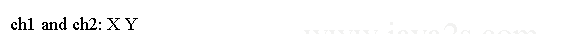`ch1` is assigned the value 88, which is the ASCII (and Unicode) value that corresponds to the letter `X`.

## Example

`char` type value can be used as an integer type and you can perform arithmetic operations.

```public class Main {
public static void main(String args[]) {
char ch1;//  ww w. j  av a2 s .  c o m

ch1 = 'X';
System.out.println("ch1 contains " + ch1);//ch1 contains X

ch1 = (char)(ch1 + 1); // increment ch1
System.out.println("ch1 is now " + ch1);//ch1 is now Y
}
}
```

The code above generates the following result.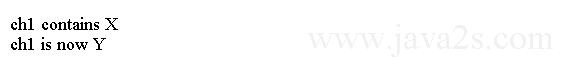## Example 2

The following code shows that we can assign non-letter character to Java char type.

```public class Main {
public static void main(String[] argv) {
char ch = 'a';
// w ww  . jav a  2 s  . com
System.out.println("ch is " + ch);//ch is a
ch = '@';

System.out.println("ch is " + ch);//ch is @
ch = '#';

System.out.println("ch is " + ch);//ch is #
ch = '\$';

System.out.println("ch is " + ch);//ch is \$
ch = '%';

System.out.println("ch is " + ch);//ch is %
}
}
```

The code above generates the following result.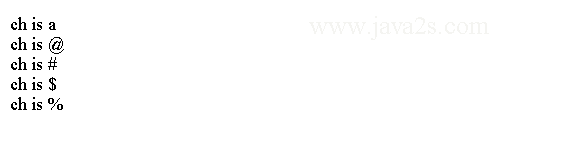## Example 3

The following code stores unicode value into a char variable. The unicode literal uses `\uxxxx` format.

```public class Main {
public static void main(String[] args) {
int x = 75;
char y = (char) x;
char half = '\u00AB';
System.out.println("y is " + y + " and half is " + half);

}
}
```

The code above generates the following result.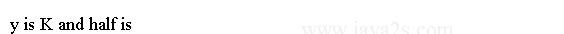## Java char value escape

The escape sequences are used to enter impossible-to-enter-directly characters.

Syntax to escape char value:

`'\''` is for the single-quote character. `'\n'` is for the newline character.

For octal notation, use the backslash followed by the three-digit number. For example, `'\141'` is the letter 'a'.

For hexadecimal, you enter a backslash-u (`\u`), then exactly four hexadecimal digits. For example, '`\u0061`' is the ISO-Latin-1 '`a`' because the top byte is zero. '`\ua432`' is a Japanese Katakana character.

```public class Main {
public static void main(String[] argv) {
char ch = '\'';

System.out.println("ch is " + ch);//ch is '

}
}
```

Character is a simple wrapper around a char.

The code above generates the following result.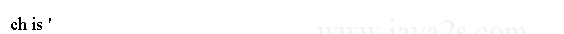## Escape value list

The following table shows the character escape sequences.

Escape SequenceDescription
\dddOctal character (ddd)